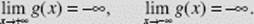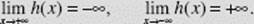﻿ ﻿DEFINITIONS AND EXAMPLES - Limits and Continuity - Calculus AB and Calculus BC

## CHAPTER 2 Limits and Continuity

Concepts and Skills

In this chapter, you will review

• general properties of limits;

• how to find limits using algebraic expressions, tables, and graphs;

• horizontal and vertical asymptotes;

• continuity;

• removable, jump, and infinite discontinuities;

• and some important theorems, including the Squeeze Theorem, the Extreme Value Theorem, and the Intermediate Value Theorem.

### A. DEFINITIONS AND EXAMPLES

The number L is the limit of the function f (x) as x approaches c if, as the values of x get arbitrarily close (but not equal) to c, the values of f (x) approach (or equal) L. We writeIn order forto exist, the values of f must tend to the same number L as x approaches c from either the left or the right. We write

One-sided limitsfor the left-hand limit of f at c (as x approaches c through values less than c), andfor the right-hand limit of f at c (as x approaches c through values greater than c).

EXAMPLE 1

The greatest-integer function g(x) = [x], shown in Figure N2–1, has different left-hand and right-hand limits at every integer. For example,This function, therefore, does not have a limit at x = 1 or, by the same reasoning, at any other integer.FIGURE N2–1

However, [x] does have a limit at every nonintegral real number. For example,EXAMPLE 2

Suppose the function y = f (x), graphed in Figure N2–2, is defined as follows:Determine whether limits of f, if any, exist at

(a) x = −2,

(b) x = 0,

(c) x = 2,

(d) x = 4.FIGURE N2–2

SOLUTIONS:

(a)so the right-hand limit exists at x = −2, even though f is not defined at x = −2.

(b)does not exist. Although f is defined at x = 0 (f (0) = 2), we observe thatwhereasFor the limit to exist at a point, the left-hand and right-hand limits must be the same.

(c)This limit exists becauseIndeed, the limit exists at x = 2 even though it is different from the value of f at 2 (f (2) = 0).

(d)so the left-hand limit exists at x = 4.

EXAMPLE 3

Prove thatSOLUTION: The graph of |x| is shown in Figure N2–3.

We examine both left- and right-hand limits of the absolute-value function as x → 0. Sinceit follows thatSince the left-hand and right-hand limits both equal 0,Note thatif c > 0 but equals −c if c < 0.FIGURE N2–3

DEFINITION

The function f (x) is said to become infinite (positively or negatively) as x approaches c if f (x) can be made arbitrarily large (positively or negatively) by taking x sufficiently close to c. We writeSince for the limit to exist it must be a finite number, neither of the preceding limits exists.

This definition can be extended to include x approaching c from the left or from the right. The following examples illustrate these definitions.

EXAMPLE 4

Describe the behavior ofnear x = 0 using limits.

SOLUTION: The graph (Figure N2–4) shows that:FIGURE N2–4

EXAMPLE 5

Describe the behavior ofnear x = 1 using limits.

SOLUTION: The graph (Figure N2–5) shows that:FIGURE N2–5

Remember that none of the limits in Examples 4 and 5 exists!

DEFINITION

We writeif the difference between f (x) and L can be made arbitrarily small by making x sufficiently large positively (or negatively).

In Examples 4 and 5, note thatEXAMPLE 6

From the graph of(Figure N2–6), describe the behavior of h using limits.

SOLUTION:FIGURE N2–6

DEFINITION

The theorems that follow in §C of this chapter confirm the conjectures made about limits of functions from their graphs.

Finally, if the function f (x) becomes infinite as x becomes infinite, then one or more of the following may hold:END BEHAVIOR OF POLYNOMIALS

Every polynomial whose degree is greater than or equal to 1 becomes infinite as x does. It becomes positively or negatively infinite, depending only on the sign of the leading coefficient and the degree of the polynomial.

EXAMPLE 7

For each function given below, describe(a) f (x) = x3 − 3x2 + 7x + 2

SOLUTION:(b) g(x) = −4x4 + 1,000,000x3 + 100

SOLUTION:(c) h(x) = −5x3 + 3x2 −4π + 8

SOLUTION:(d) k(x) = π − 0.001x

SOLUTION:It’s easy to write rules for the behavior of a polynomial as x becomes infinite!

﻿